Question

# A student determined the molar volume of CO2 gas using the procedure described in this experiment?...

A student determined the molar volume of CO2 gas using the procedure described in this experiment?

He collected the following data.

mass of empty Mylar balloon and fastener,g 3.03
mass of Mylar balloon, fastener, and CO2 gas, g 3.99
volume (capacity) of Mylar balloon, mL 1730
barometric pressure, in. Hg = 28.96
laboratory temperature, C = 22

a)express the pressure in atmospheres. [Note: 1 atm = 29.92in. Hg]

b)express the temperature in Kelvin

c)express the volume of the balloon in liters

d)determine the number of moles of CO2 in the inflated balloon

e)determine the mass of air displaced by the inflated balloon

f)determine the measured mass of the CO2 in the balloon

g)determine the actual mass of the CO2 in the balloon

h)determine the molar mass of CO2

i)determine the percent error in your calculated molar mass for CO2, based on the theoretical value of 44.01 g/mol.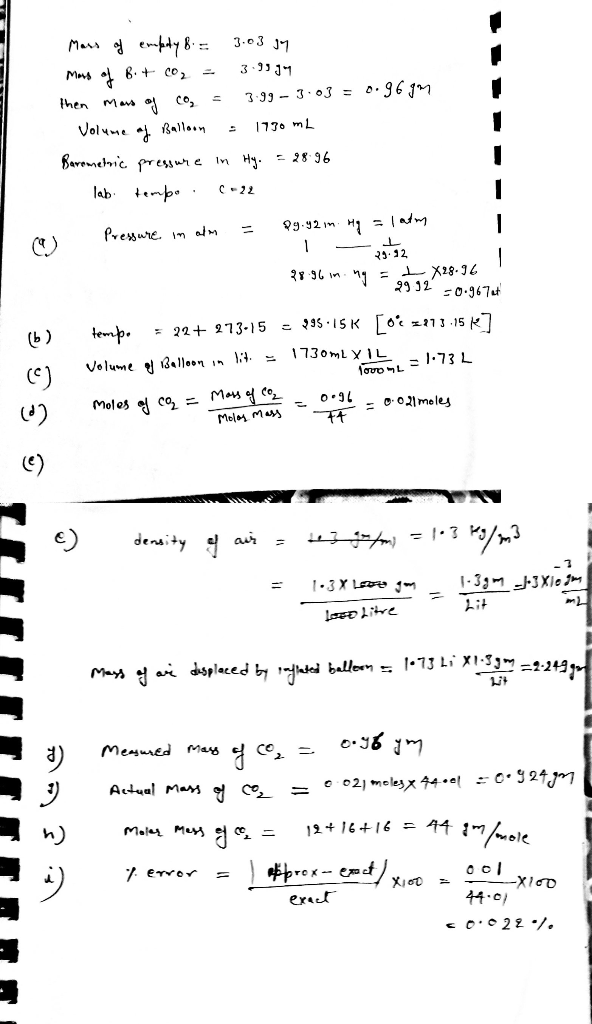#### Earn Coins

Coins can be redeemed for fabulous gifts.

Similar Homework Help Questions
• ### 132 PROPOS22: Determining the Molar Mass of Co2 e) Determine the mass of air displaced by...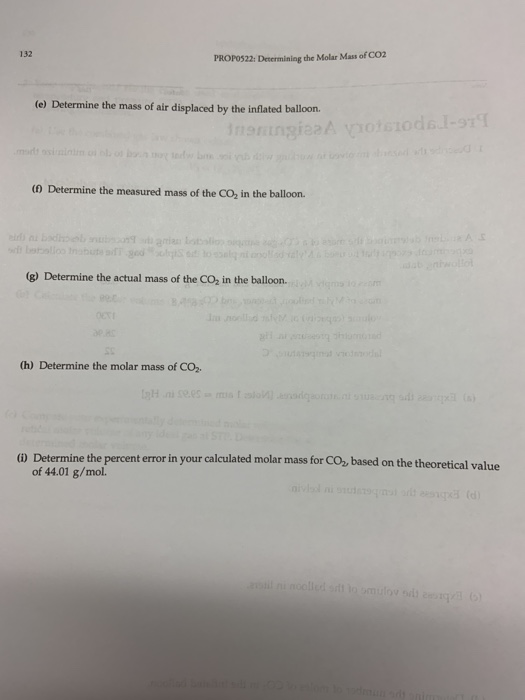132 PROPOS22: Determining the Molar Mass of Co2 e) Determine the mass of air displaced by the inflated balloorn (0 Determine the measured mass of the CO2 in the balloon. (g) Determine the actual mass of the CO2 in the balloon. (h) Determine the molar mass of Co, () Determine the percent error in your calculated molar mass for CO2, based on the theoretical value of 44.01 g/mol. eriment, except following data: e mass of a CO2 gas sample collected...

• ### A-D A student performed an experiment in which they collected hydrogen gas from the reaction of...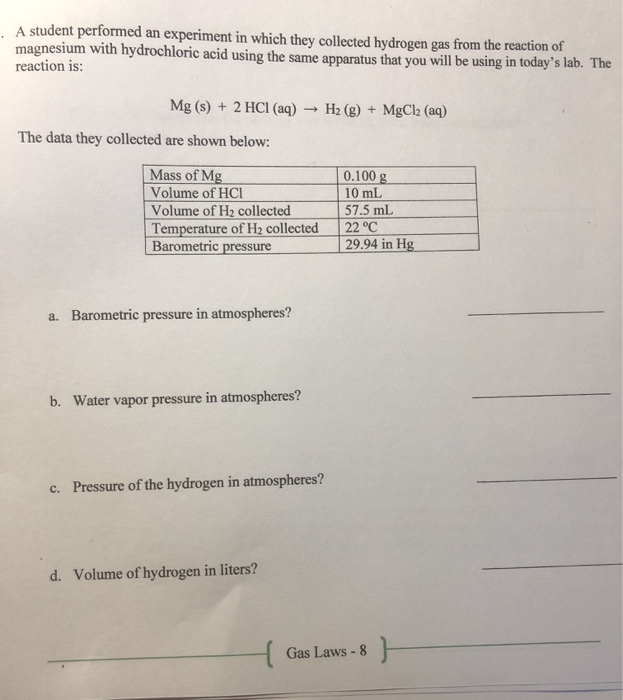A-D A student performed an experiment in which they collected hydrogen gas from the reaction of magnesium with hydrochloric acid using the same apparatus that you will be using in today's lab. The reaction is: Mg(s) + 2 HCl(aq) → H2(g) + MgCl2 (aq) The data they collected are shown below: Mass of Mg Volume of HCI Volume of H2 collected Temperature of H2 collected Barometric pressure 0.100 g 10 mL 57.5 mL 22 °C 29.94 in Hg a. Barometric...

• ### A student follows the procedure described in the experiment and collected the following data: 25.5 mL...

A student follows the procedure described in the experiment and collected the following data: 25.5 mL of hydrogen gas collected, barometric pressure of 755 mm Hg, and a temperature of 298 K. Use the ideal gas law (PV=nRT) to answer the questions below. The R value is 0.08206 L atm / Kmol and our data must be in these units. The temperature is given in Kelvin, so no conversion is needed. But the hydrogen gas volume and pressure need conversion...

• ### A student performed an experiment in which hydrogen gas was collected over water from the reaction...

A student performed an experiment in which hydrogen gas was collected over water from the reaction of magnesium and hydrochloric acid using the apparatus shown and accoridng to the reaction equation and the data collected shown below: Mass of Mg 0.100 g Volume of HCl 10 mL Volume of H2 collected 57.5 mL Temperature of H2 collected 22.0 oC Barometric pressure 29.94 inHg Mg(s) + 2 HCl(aq) → H2(g) + MgCl2(aq)                 a. Barometric pressure in atmospheres? b. Water vapor pressure...

• ### Use the data table to answer the questions please. Thank you in advance! :) Gas Laws...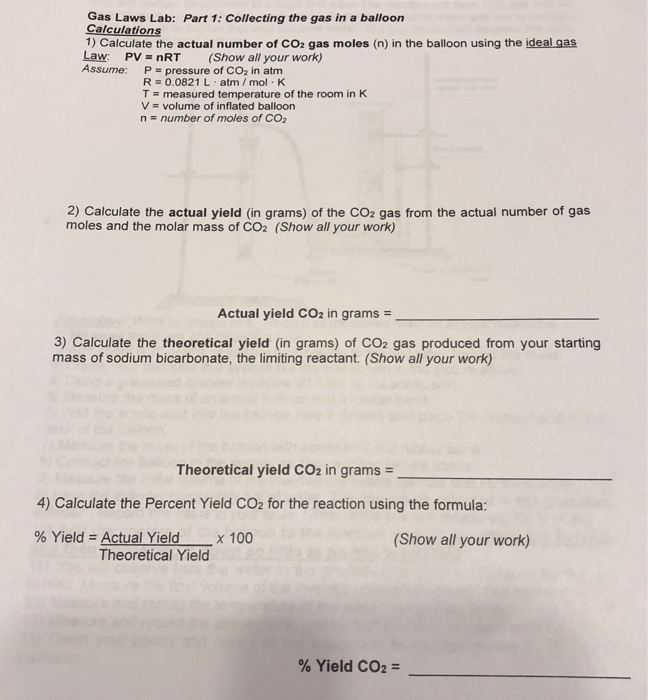Use the data table to answer the questions please. Thank you in advance! :) Gas Laws Lab: Part 1: Collecting the gas in a balloon Calculations 1) Calculate the actual number of CO2 gas moles (n) in the balloon using the ideal gas Law PV= nRT (Show all your work) Assume: P pressure of CO2 in atm R 0.0821 L atm/ mol K T measured temperature of the room in K V volume of inflated balloon n number of moles...

• ### What is the new volume, in liters, of a 25.0 L sample of air at 843.6...

What is the new volume, in liters, of a 25.0 L sample of air at 843.6 mmHg and 152°C that is compressed and cooled to 35°C and 1520 mmHg? 2.. A balloon containing 7.83 moles of He has a volume of 27.5 L at a certain temperature and pressure. What would be the volume of the balloon, in liters, if 1.00 mole of Heis removed from the balloon at constant temperature and pressure? 3. Calculate the pressure in atmospheres, of...

• ### atm 4 Mass of benker and displaced water 368.910 S Mass of empty beaker 144.502 Mass...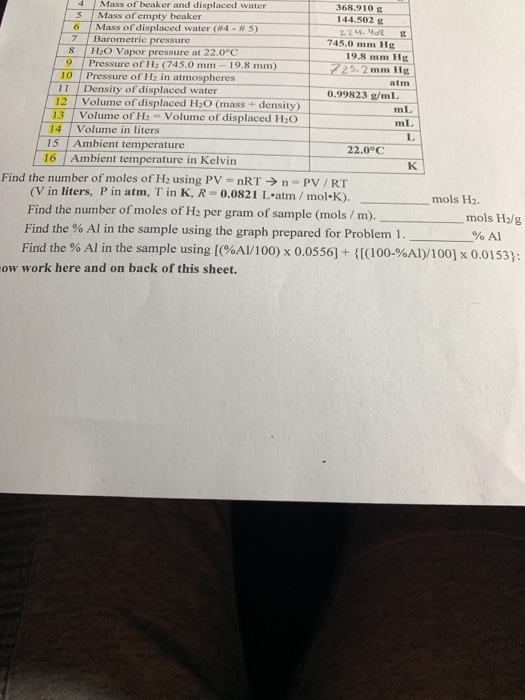atm 4 Mass of benker and displaced water 368.910 S Mass of empty beaker 144.502 Mass of displaced water (W4 - N5) 224. MRE 7 Barometric pressure 745,mm Hg HO Vapor pressure at 22.0°C 19.8 mm Hg Pressure of H2 (745.0 mm - 19.8 mm) 725.2 mm Hg 10 Pressure of Hy in atmospheres Density of displaced water 0.99823 mL Volume of displaced H2O (mass + density) Volume of H2 - Volume of displaced H2O mL Volume in liters 15...

• ### NTERACTIVE EXAMPLE General Gas Law Helium-filled balloons are used to carry scientific instruments high into the...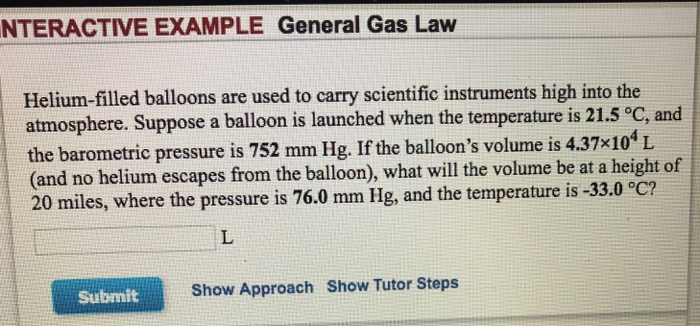NTERACTIVE EXAMPLE General Gas Law Helium-filled balloons are used to carry scientific instruments high into the atmosphere. Suppose a balloon is launched when the temperature is 21.5 °C, and the barometric pressure is 752 mm Hg. If the balloon's volume is 4.37x10L (and no helium escapes from the balloon), what will the volume be at a height of 20 miles, where the pressure is 76.0 mm Hg, and the temperature is -33.0 °C? Submit Show Approach Show Tutor Steps TOR...

• ### 8 The plot of NH2 vs. % Al will be a straight line. To fix the...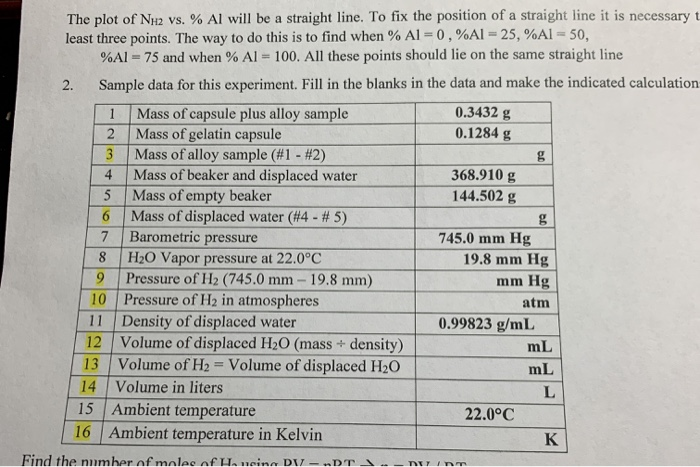8 The plot of NH2 vs. % Al will be a straight line. To fix the position of a straight line it is necessary t least three points. The way to do this is to find when % AL=0,%AI - 25,%AI -50. %Al = 75 and when % Al = 100. All these points should lie on the same straight line 2. Sample data for this experiment. Fill in the blanks in the data and make the indicated calculation 1...

• ### A student performed the experiment described in this module. The 5.00-mL mass of the 2.15% percent...

A student performed the experiment described in this module. The 5.00-mL mass of the 2.15% percent by mass H2O2 solution used was 5.03 g. The water temperature was 230C, and the barometric pressure was 31.2 in. Hg. After the student immersed the yeast in the peroxide solution, she observed a 38.60-mL volume change in system volume. (1) Convert the barometric pressure to torr. (2) Obtain the water vapour pressure at the water temperature. (3) Calculate the pressure, in torr, exerted...